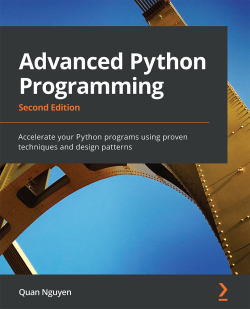# Advanced Python Programming - Second Edition

By Quan Nguyen
• \$51.99 Print
• \$41.99 eBook
• \$12.99 eBook + Subscription
What do you get with a Packt Subscription?

• Constantly updated with 100+ new titles each month
• Breadth and depth in over 1,000+ technologies

Python's powerful capabilities for implementing robust and efficient programs make it one of the most sought-after programming languages.

In this book, you'll explore the tools that allow you to improve performance and take your Python programs to the next level.

This book starts by examining the built-in as well as external libraries that streamline tasks in the development cycle, such as benchmarking, profiling, and optimizing. You'll then get to grips with using specialized tools such as dedicated libraries and compilers to increase your performance at number-crunching tasks, including training machine learning models.

The book covers concurrency, a major solution to making programs more efficient and scalable, and various concurrent programming techniques such as multithreading, multiprocessing, and asynchronous programming.

You'll also understand the common problems that cause undesirable behavior in concurrent programs.

Finally, you'll work with a wide range of design patterns, including creational, structural, and behavioral patterns that enable you to tackle complex design and architecture challenges, making your programs more robust and maintainable.

By the end of the book, you'll be exposed to a wide range of advanced functionalities in Python and be equipped with the practical knowledge needed to apply them to your use cases.

Publication date:
March 2022
Publisher
Packt
Pages
606
ISBN
9781801814010

# Chapter 1: Benchmarking and Profiling

Recognizing the slow parts of your program is the single most important task when it comes to speeding up your code. In most cases, the code that causes the application to slow down is a very small fraction of the program. By identifying these critical sections, you can focus on the parts that need the most improvement without wasting time in micro-optimization.

Profiling is a technique that allows us to pinpoint the most resource-intensive parts of an application. A profiler is a program that runs an application and monitors how long each function takes to execute, thus detecting the functions on which your application spends most of its time.

Python provides several tools to help us find these bottlenecks and measure important performance metrics. In this chapter, we will learn how to use the standard `cProfile` module and the `line_profiler` third-party package. We will also learn how to profile the memory consumption of an application through the `memory_profiler` tool. Another useful tool that we will cover is KCachegrind, which can be used to graphically display the data produced by various profilers.

Finally, benchmarks are small scripts used to assess the total execution time of your application. We will learn how to write benchmarks and use them to accurately time your programs.

The topics we will cover in this chapter are listed here:

• Writing tests and benchmarks
• Writing better tests and benchmarks with `pytest-benchmark`
• Finding bottlenecks with `cProfile`
• Optimizing our code
• Using the `dis` module
• Profiling memory usage with `memory_profiler`

By the end of the chapter, you will have gained a solid understanding of how to optimize a Python program and will be armed with practical tools that facilitate the optimization process.

# Technical requirements

To follow the content of this chapter, you should have a basic understanding of Python programming and be familiar with core concepts such as variables, classes, and functions. You should also be comfortable with working with the command line to run Python programs. Finally, the code for this chapter can be found in the following GitHub repository: https://github.com/PacktPublishing/Advanced-Python-Programming-Second-Edition/tree/main/Chapter01.

In the early development stages, the design of a program can change quickly and may require large rewrites and reorganizations of the code base. By testing different prototypes without the burden of optimization, you are free to devote your time and energy to ensure that the program produces correct results and that the design is flexible. After all, who needs an application that runs fast but gives the wrong answer?

The mantras that you should remember when optimizing your code are outlined here:

• Make it run: We have to get the software in a working state and ensure that it produces the correct results. This exploratory phase serves to better understand the application and to spot major design issues in the early stages.
• Make it right: We want to ensure that the design of the program is solid. Refactoring should be done before attempting any performance optimization. This really helps separate the application into independent and cohesive units that are easy to maintain.
• Make it fast: Once our program is working and well structured, we can focus on performance optimization. We may also want to optimize memory usage if that constitutes an issue.

In this section, we will write and profile a particle simulator test application. A simulator is a program that considers some particles and simulates their movement over time according to a set of laws that we impose. These particles can be abstract entities or correspond to physical objects—for example, billiard balls moving on a table, molecules in a gas, stars moving through space, smoke particles, fluids in a chamber, and so on.

## Building a particle simulator

Computer simulations are useful in fields such as physics, chemistry, astronomy, and many other disciplines. The applications used to simulate systems are particularly performance-intensive, and scientists and engineers spend an inordinate amount of time optimizing their code. In order to study realistic systems, it is often necessary to simulate a very high number of bodies, and every small increase in performance counts.

In our first example, we will simulate a system containing particles that constantly rotate around a central point at various speeds, just like the hands of a clock.

The necessary information to run our simulation will be the starting positions of the particles, the speed, and the rotation direction. From these elements, we have to calculate the position of the particle in the next instant of time. An example system is shown in the following diagram. The origin of the system is the `(0, 0)` point, the position is indicated by the x, y vector, and the velocity is indicated by the vx, vy vector: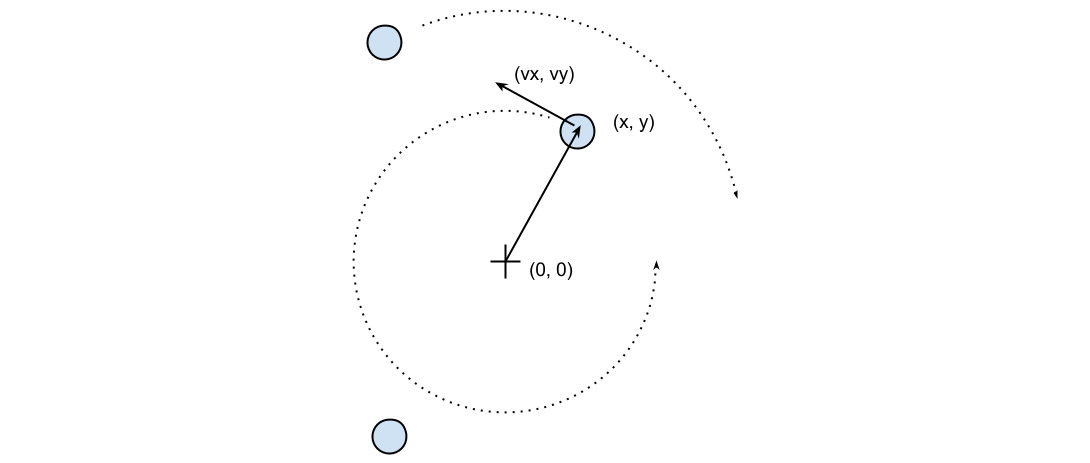Figure 1.1 – An example of a particle system

The basic feature of a circular motion is that the particles always move perpendicular to the direction connecting the particle and the center. To move the particle, we simply change the position by taking a series of very small steps (which correspond to advancing the system for a small interval of time) in the direction of motion, as shown in the following diagram:Figure 1.2 – Movement of a particle

We will start by designing the application in an object-oriented (OO) way. According to our requirements, it is natural to have a generic `Particle` class that stores the particle positions, `x` and `y`, and their angular velocity, `ang_vel`, as illustrated in the following code snippet:

```    class Particle:
def __init__(self, x, y, ang_vel):
self.x = x
self.y = y
self.ang_vel = ang_vel```

Note that we accept positive and negative numbers for all the parameters (the sign of `ang_vel` will simply determine the direction of rotation).

Another class, called `ParticleSimulator`, will encapsulate the laws of motion and will be responsible for changing the positions of the particles over time. The `__init__` method will store a list of `Particle` instances, and the `evolve` method will change the particle positions according to our laws. The code is illustrated in the following snippet:

```class ParticleSimulator:
def __init__(self, particles):
self.particles = particles```

We want the particles to rotate around the position corresponding to the `x=0` and `y=0` coordinates, at a constant speed. The direction of the particles will always be perpendicular to the direction from the center (refer to Figure 1.1 in this chapter). To find the direction of the movement along the x and y axes (corresponding to the Python `v_x` and `v_y` variables), it is sufficient to use these formulae:

```    v_x = -y / (x**2 + y**2)**0.5
v_y = x / (x**2 + y**2)**0.5```

If we let one of our particles move, after a certain time t, it will reach another position following a circular path. We can approximate a circular trajectory by dividing the time interval, t, into tiny time steps, dt, where the particle moves in a straight line tangentially to the circle. (Note that higher-order curves could be used rather than straight lines for better accuracy, but we will stick with lines as the simplest approximation.) The final result is just an approximation of a circular motion.

In order to avoid a strong divergence, such as the one illustrated in the following diagram, it is necessary to take very small time steps: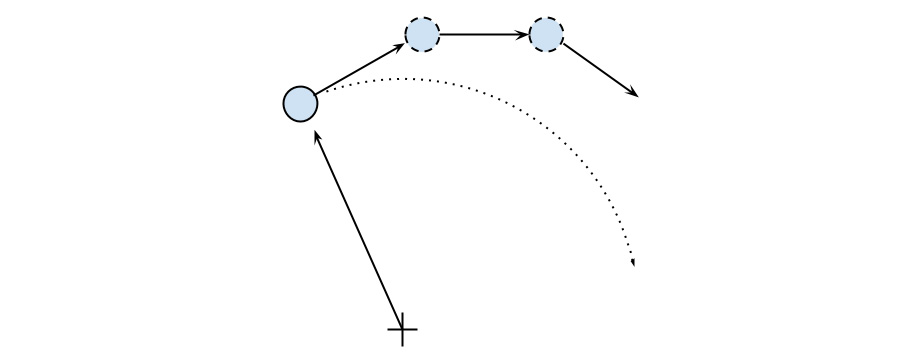Figure 1.3 – Undesired divergence in particle motion due to large time steps

In a more schematic way, we have to carry out the following steps to calculate the particle position at time t:

1. Calculate the direction of motion (`v_x` and `v_y`).
2. Calculate the displacement (`d_x` and `d_y`), which is the product of the time step, angular velocity, and direction of motion.
3. Repeat Steps 1 and 2 enough times to cover the total time t.

The following code snippet shows the full `ParticleSimulator` implementation:

```    class ParticleSimulator:
def __init__(self, particles):
self.particles = particles
def evolve(self, dt):
timestep = 0.00001
nsteps = int(dt/timestep)

for i in range(nsteps):
for p in self.particles:
# 1. calculate the direction
norm = (p.x**2 + p.y**2)**0.5
v_x = -p.y/norm
v_y = p.x/norm
# 2. calculate the displacement
d_x = timestep * p.ang_vel * v_x
d_y = timestep * p.ang_vel * v_y
p.x += d_x
p.y += d_y
# 3. repeat for all the time steps```

And with that, we have finished building the foundation of our particle simulator. Next, we will see it in action by visualizing the simulated particles.

## Visualizing the simulation

We can use the `matplotlib` library here to visualize our particles. This library is not included in the Python standard library, but it can be easily installed using the `pip install matplotlib` command.

Alternatively, you can use the Anaconda Python distribution (https://store.continuum.io/cshop/anaconda/), which includes `matplotlib` and most of the other third-party packages used in this book. Anaconda is free and is available for Linux, Windows, and Mac.

To make an interactive visualization, we will use the `matplotlib.pyplot.plot` function to display the particles as points and the `matplotlib.animation.FuncAnimation` class to animate the evolution of the particles over time.

The `visualize` function takes a `ParticleSimulator` particle instance as an argument and displays the trajectory in an animated plot. The steps necessary to display the particle trajectory using the `matplotlib` tools are outlined here:

1. Set up the axes and use the `plot` function to display the particles. The `plot` function takes a list of x and y coordinates.
2. Write an initialization function, `init`, and a function, `animate`, that updates the x and y coordinates using the `line.set_data` method. Note that in `init`, we need to return the line data in the form of `line`, due to syntactic reasons.
3. Create a `FuncAnimation` instance by passing the `init` and `animate` functions and the `interval` parameters, which specify the update interval, and `blit`, which improves the update rate of the image.
4. Run the animation with `plt.show()`, as illustrated in the following code snippet:
```    from matplotlib import pyplot as plt
from matplotlib import animation
def visualize(simulator):
X = [p.x for p in simulator.particles]
Y = [p.y for p in simulator.particles]
fig = plt.figure()
ax = plt.subplot(111, aspect='equal')
line, = ax.plot(X, Y, 'ro')

# Axis limits
plt.xlim(-1, 1)
plt.ylim(-1, 1)
# It will be run when the animation starts
def init():
line.set_data([], [])
return line, # The comma is important!
def animate(i):
# We let the particle evolve for 0.01 time
units
simulator.evolve(0.01)
X = [p.x for p in simulator.particles]
Y = [p.y for p in simulator.particles]
line.set_data(X, Y)
return line,
# Call the animate function each 10 ms
anim = animation.FuncAnimation(fig,
animate,init_func=init,blit=True,
interval=10)
plt.show()```

To test this code, we define a small function, `test_visualize`, that animates a system of three particles rotating in different directions. Note in the following code snippet that the third particle completes a round three times faster than the others:

```    def test_visualize():
particles = [
Particle(0.3, 0.5, 1),
Particle(0.0, -0.5, -1),
Particle(-0.1, -0.4, 3)
]
simulator = ParticleSimulator(particles)
visualize(simulator)
if __name__ == '__main__':
test_visualize()```

The `test_visualize` function is helpful to graphically understand the system time evolution. Simply close the animation window when you'd like to terminate the program. With this program in hand, in the following section, we will write more test functions to properly verify program correctness and measure performance.

# Writing tests and benchmarks

Now that we have a working simulator, we can start measuring our performance and tune up our code so that the simulator can handle as many particles as possible. As a first step, we will write a test and a benchmark.

We need a test that checks whether the results produced by the simulation are correct or not. Optimizing a program commonly requires employing multiple strategies; as we rewrite our code multiple times, bugs may easily be introduced. A solid test suite ensures that the implementation is correct at every iteration so that we are free to go wild and try different things with the confidence that, if the test suite passes, the code will still work as expected. More specifically, what we are implementing here are called unit tests, which aim to verify the intended logic of the program regardless of the implementation details, which may change during optimization.

Our test will take three particles, simulate them for `0.1` time units, and compare the results with those from a reference implementation. A good way to organize your tests is using a separate function for each different aspect (or unit) of your application. Since our current functionality is included in the `evolve` method, our function will be named `test_evolve`. The following code snippet shows the `test_evolve` implementation. Note that, in this case, we compare floating-point numbers up to a certain precision through the `fequal` function:

```    def test_evolve():
particles = [Particle( 0.3,  0.5, +1),
Particle( 0.0, -0.5, -1),
Particle(-0.1, -0.4, +3)
]
simulator = ParticleSimulator(particles)
simulator.evolve(0.1)
p0, p1, p2 = particles
def fequal(a, b, eps=1e-5):
return abs(a - b) < eps
assert fequal(p0.x, 0.210269)
assert fequal(p0.y, 0.543863)
assert fequal(p1.x, -0.099334)
assert fequal(p1.y, -0.490034)
assert fequal(p2.x,  0.191358)
assert fequal(p2.y, -0.365227)
if __name__ == '__main__':
test_evolve()```

The `assert` statements will raise an error if the included conditions are not satisfied. Upon running the `test_evolve` function, if you notice no error or output printed out, that means all the conditions are met.

A test ensures the correctness of our functionality but gives little information about its running time. A benchmark is a simple and representative use case that can be run to assess the running time of an application. Benchmarks are very useful to keep score of how fast our program is with each new version that we implement.

We can write a representative benchmark by instantiating a thousand `Particle` objects with random coordinates and angular velocity and feeding them to a `ParticleSimulator` class. We then let the system evolve for `0.1` time units. The code is illustrated in the following snippet:

```    from random import uniform
def benchmark():
particles = [
Particle(uniform(-1.0, 1.0), uniform(-1.0, 1.0),
uniform(-1.0, 1.0))
for i in range(1000)]
simulator = ParticleSimulator(particles)
simulator.evolve(0.1)
if __name__ == '__main__':
benchmark()```

With the benchmark program implemented, we now need to run it and keep track of the time needed for the benchmark to complete execution, which we will see next. (Note that when you run these tests and benchmarks on your own system, you are likely to see different numbers listed in the text, which is completely normal and dependent on your system configurations and Python version.)

A very simple way to time a benchmark is through the Unix `time` command. Using the `time` command, as follows, you can easily measure the execution time of an arbitrary process:

```    \$ time python simul.py
real    0m1.051s
user    0m1.022s
sys     0m0.028s```

The `time` command is not available for Windows. To install Unix tools such as `time` on Windows, you can use the `cygwin` shell, downloadable from the official website (http://www.cygwin.com/). Alternatively, you can use similar PowerShell commands, such as `Measure-Command` (https://msdn.microsoft.com/en-us/powershell/reference/5.1/microsoft.powershell.utility/measure-command), to measure execution time.

By default, `time` displays three metrics, as outlined here:

• `real`: The actual time spent running the process from start to finish, as if it were measured by a human with a stopwatch.
• `user`: The cumulative time spent by all the central processing units (CPUs) during the computation.
• `sys`: The cumulative time spent by all the CPUs during system-related tasks, such as memory allocation.

Note

Sometimes, `user` and `sys` might be greater than `real`, as multiple processors may work in parallel.

`time` also offers richer formatting options. For an overview, you can explore its manual (using the `man time` command). If you want a summary of all the metrics available, you can use the `-v` option.

The Unix `time` command is one of the simplest and most direct ways to benchmark a program. For an accurate measurement, the benchmark should be designed to have a long enough execution time (in the order of seconds) so that the setup and teardown of the process are small compared to the execution time of the application. The `user` metric is suitable as a monitor for the CPU performance, while the `real` metric also includes the time spent on other processes while waiting for input/output (I/O) operations.

Another convenient way to time Python scripts is the `timeit` module. This module runs a snippet of code in a loop for n times and measures the total execution time. Then, it repeats the same operation r times (by default, the value of r is `3`) and records the time of the best run. Due to this timing scheme, `timeit` is an appropriate tool to accurately time small statements in isolation.

The `timeit` module can be used as a Python package, from the command line or from IPython.

IPython is a Python shell design that improves the interactivity of the Python interpreter. It boosts tab completion and many utilities to time, profile, and debug your code. We will use this shell to try out snippets throughout the book. The IPython shell accepts magic commands—statements that start with a `%` symbol—that enhance the shell with special behaviors. Commands that start with `%%` are called cell magics, which can be applied on multiline snippets (termed as cells).

IPython is available on most Linux distributions through `pip` and is included in Anaconda. You can use IPython as a regular Python shell (`ipython`), but it is also available in a Qt-based version (`ipython qtconsole`) and as a powerful browser-based interface (`jupyter notebook`).

In IPython and command-line interfaces (CLIs), it is possible to specify the number of loops or repetitions with the `-n` and `-r` options. If not specified, they will be automatically inferred by `timeit`. When invoking `timeit` from the command line, you can also pass some setup code, through the `-s` option, which will execute before the benchmark. In the following snippet, the `IPython` command line and Python module version of `timeit` are demonstrated:

```# IPython Interface
\$ ipython
In : from simul import benchmark
In : %timeit benchmark()
1 loops, best of 3: 782 ms per loop
# Command Line Interface
\$ python -m timeit -s 'from simul import benchmark'
'benchmark()'
10 loops, best of 3: 826 msec per loop
# Python Interface
# put this function into the simul.py script
import timeit
result = timeit.timeit('benchmark()',
setup='from __main__ import benchmark', number=10)
# result is the time (in seconds) to run the whole loop
result = timeit.repeat('benchmark()',
setup='from __main__ import benchmark', number=10, \
repeat=3)
# result is a list containing the time of each repetition
(repeat=3 in this case)```

Note that while the command line and IPython interfaces automatically infer a reasonable number of loops `n`, the Python interface requires you to explicitly specify a value through the `number` argument.

# Writing better tests and benchmarks with pytest-benchmark

The Unix `time` command is a versatile tool that can be used to assess the running time of small programs on a variety of platforms. For larger Python applications and libraries, a more comprehensive solution that deals with both testing and benchmarking is `pytest`, in combination with its `pytest-benchmark` plugin.

In this section, we will write a simple benchmark for our application using the `pytest` testing framework. For those who are, the `pytest` documentation, which can be found at http://doc.pytest.org/en/latest/, is the best resource to learn more about the framework and its uses.

You can install `pytest` from the console using the `pip install pytest` command. The benchmarking plugin can be installed, similarly, by issuing the `pip install pytest-benchmark` command.

A testing framework is a set of tools that simplifies writing, executing, and debugging tests, and provides rich reports and summaries of the test results. When using the `pytest` framework, it is recommended to place tests separately from the application code. In the following example, we create a `test_simul.py` file that contains the `test_evolve` function:

```    from simul import Particle, ParticleSimulator
def test_evolve():
particles = [
Particle( 0.3,  0.5, +1),
Particle( 0.0, -0.5, -1),
Particle(-0.1, -0.4, +3)
]
simulator = ParticleSimulator(particles)
simulator.evolve(0.1)

p0, p1, p2 = particles
def fequal(a, b, eps=1e-5):
return abs(a - b) < eps
assert fequal(p0.x, 0.210269)
assert fequal(p0.y, 0.543863)
assert fequal(p1.x, -0.099334)
assert fequal(p1.y, -0.490034)
assert fequal(p2.x,  0.191358)
assert fequal(p2.y, -0.365227)```

The `pytest` executable can be used from the command line to discover and run tests contained in Python modules. To execute a specific test, we can use the `pytest path/to/module.py::function_name` syntax. To execute `test_evolve`, we can type the following command in a console to obtain simple but informative output:

```\$ pytest test_simul.py::test_evolve
platform linux -- Python 3.5.2, pytest-3.0.5, py-1.4.32,
pluggy-0.4.0
rootdir: /home/gabriele/workspace/hiperf/chapter1, inifile:
plugins:
collected 2 items
test_simul.py .
=========================== 1 passed in 0.43 seconds ===========================```

Once we have a test in place, it is possible for you to execute your test as a benchmark using the `pytest-benchmark` plugin. If we change our `test` function so that it accepts an argument named `benchmark`, the `pytest` framework will automatically pass the `benchmark` resource as an argument (in `pytest` terminology, these resources are called fixtures). The `benchmark` resource can be called by passing the function that we intend to benchmark as the first argument, followed by the additional arguments. In the following snippet, we illustrate the edits necessary to benchmark the `ParticleSimulator.evolve` function:

```    from simul import Particle, ParticleSimulator
def test_evolve(benchmark):
# ... previous code
benchmark(simulator.evolve, 0.1)```

To run the benchmark, it is sufficient to rerun the `pytest test_simul.py::test_evolve` command. The resulting output will contain detailed timing information regarding the `test_evolve` function, as shown here: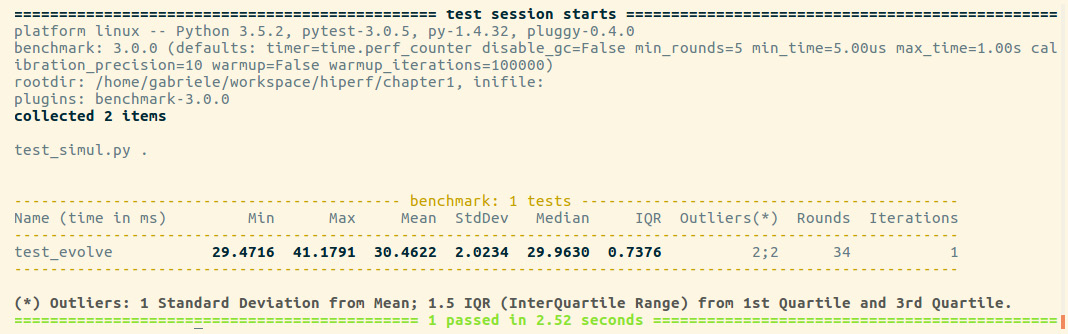Figure 1.4 – Output from pytest

For each test collected, `pytest-benchmark` will execute the `benchmark` function several times and provide a statistic summary of its running time. The preceding output shown is very interesting as it shows how running times vary between runs.

In this example, the benchmark in `test_evolve` was run `34` times (`Rounds` column), its timings ranged between `29` and `41` milliseconds (ms) (`Min` and `Max`), and the `Average` and `Median` times were fairly similar at about `30` ms, which is actually very close to the best timing obtained. This example demonstrates how there can be substantial performance variability between runs and that, as opposed to taking timings with one-shot tools such as `time`, it is a good idea to run the program multiple times and record a representative value, such as the minimum or the median.

`pytest-benchmark` has many more features and options that can be used to take accurate timings and analyze the results. For more information, consult the documentation at http://pytest-benchmark.readthedocs.io/en/stable/usage.html.

# Finding bottlenecks with cProfile

After assessing the correctness and timing of the execution time of the program, we are ready to identify the parts of the code that need to be tuned for performance. We typically aim to identify parts that are small compared to the size of the program.

Two profiling modules are available through the Python standard library, as outlined here:

• The profile module: This module is written in pure Python and adds significant overhead to the program execution. Its presence in the standard library is due to its vast platform support and the ease with which it can be extended.
• The cProfile module: This is the main profiling module, with an interface equivalent to `profile`. It is written in C, has a small overhead, and is suitable as a general-purpose profiler.

The `cProfile` module can be used in three different ways, as follows:

• From the command line
• As a Python module
• With IPython

`cProfile` does not require any change in the source code and can be executed directly on an existing Python script or function. You can use `cProfile` from the command line in this way:

`\$ python -m cProfile simul.py`

This will print a long output containing several profiling metrics of all of the functions called in the application. You can use the `-s` option to sort the output by a specific metric. In the following snippet, the output is sorted by the `tottime` metric, which will be described here:

`\$ python -m cProfile -s tottime simul.py`

The data produced by `cProfile` can be saved in an output file by passing the `-o` option. The format that `cProfile` uses is readable by the `stats` module and other tools. The usage of the`-o` option is shown here:

`\$ python -m cProfile -o prof.out simul.py`

The usage of `cProfile` as a Python module requires invoking the `cProfile.run` function in the following way:

```    from simul import benchmark
import cProfile
cProfile.run("benchmark()")```

You can also wrap a section of code between method calls of a `cProfile.Profile` object, as shown here:

```    from simul import benchmark
import cProfile
pr = cProfile.Profile()
pr.enable()
benchmark()
pr.disable()
pr.print_stats()```

`cProfile` can also be used interactively with IPython. The `%prun` magic command lets you profile an individual function call, as illustrated in the following screenshot: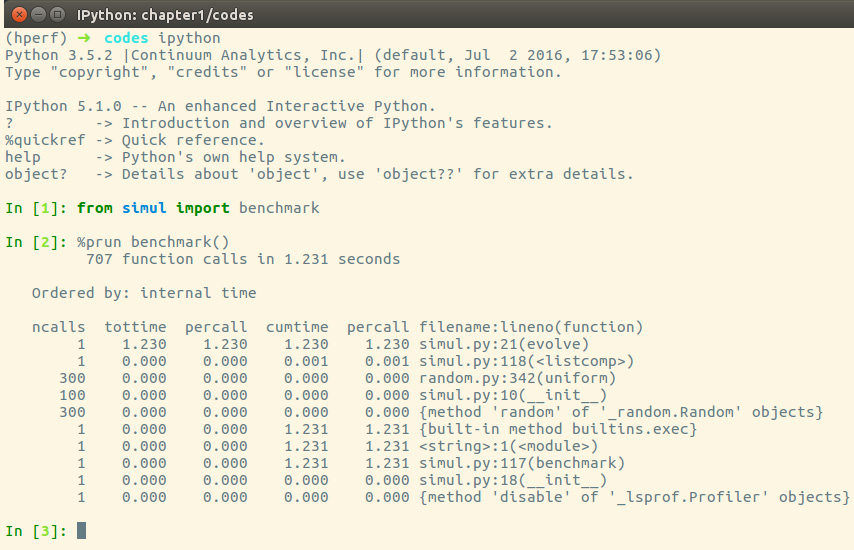Figure 1.5 – Using cProfile within IPython

The `cProfile` output is divided into five columns, as follows:

• `ncalls`: The number of times the function was called.
• `tottime`: The total time spent in the function without taking into account the calls to other functions.
• `cumtime`: The time spent in the function including other function calls.
• `percall`: The time spent for a single call of the function—this can be obtained by dividing the total or cumulative time by the number of calls.
• `filename:lineno`: The filename and corresponding line numbers. This information is not available when calling C extension modules.

The most important metric is `tottime`, the actual time spent in the function body excluding subcalls, which tells us exactly where the bottleneck is.

Unsurprisingly, the largest portion of time is spent in the `evolve` function. We can imagine that the loop is the section of the code that needs performance tuning. `cProfile` only provides information at the function level and does not tell us which specific statements are responsible for the bottleneck. Fortunately, as we will see in the next section, the `line_profiler` tool is capable of providing line-by-line information of the time spent in the function.

Analyzing the `cProfile` text output can be daunting for big programs with a lot of calls and subcalls. Some visual tools aid the task by improving navigation with an interactive, graphical interface.

## Graphically analyzing profiling results

KCachegrind is a graphical user interface (GUI) useful for analyzing the profiling output emitted by `cProfile`.

KCachegrind is available in the Ubuntu 16.04 official repositories. The Qt port, QCacheGrind, can be downloaded for Windows from http://sourceforge.net/projects/qcachegrindwin/. Mac users can compile QCacheGrind using MacPorts (http://www.macports.org/) by following the instructions present in the blog post at http://blogs.perl.org/users/rurban/2013/04/install-kachegrind-on-macosx-with-ports.html.

KCachegrind can't directly read the output files produced by `cProfile`. Luckily, the `pyprof2calltree` third-party Python module is able to convert the `cProfile` output file into a format readable by KCachegrind.

You can install `pyprof2calltree` from the Python Package Index (PyPI) using the `pip install pyprof2calltree` command.

To best show the KCachegrind features, we will use another example with a more diversified structure. We define a recursive function, `factorial`, and two other functions that use `factorial`, named `taylor_exp` and `taylor_sin`. They represent the polynomial coefficients of the Taylor approximations of `exp(x)` and `sin(x)` and are illustrated in the following code snippet:

```    def factorial(n):
if n == 0:
return 1.0
else:
return n * factorial(n-1)
def taylor_exp(n):
return [1.0/factorial(i) for i in range(n)]
def taylor_sin(n):
res = []
for i in range(n):
if i % 2 == 1:
res.append((-1)**((i-1)/2)/
float(factorial(i)))
else:
res.append(0.0)
return res
def benchmark():
taylor_exp(500)
taylor_sin(500)
if __name__ == '__main__':
benchmark()```

To access profile information, we first need to generate a `cProfile` output file, as follows:

`\$ python -m cProfile -o prof.out taylor.py`

Then, we can convert the output file with `pyprof2calltree` and launch KCachegrind by running the following code:

```\$ pyprof2calltree -i prof.out -o prof.calltree
\$ kcachegrind prof.calltree # or qcachegrind prof.calltree```

The output is shown in the following screenshot: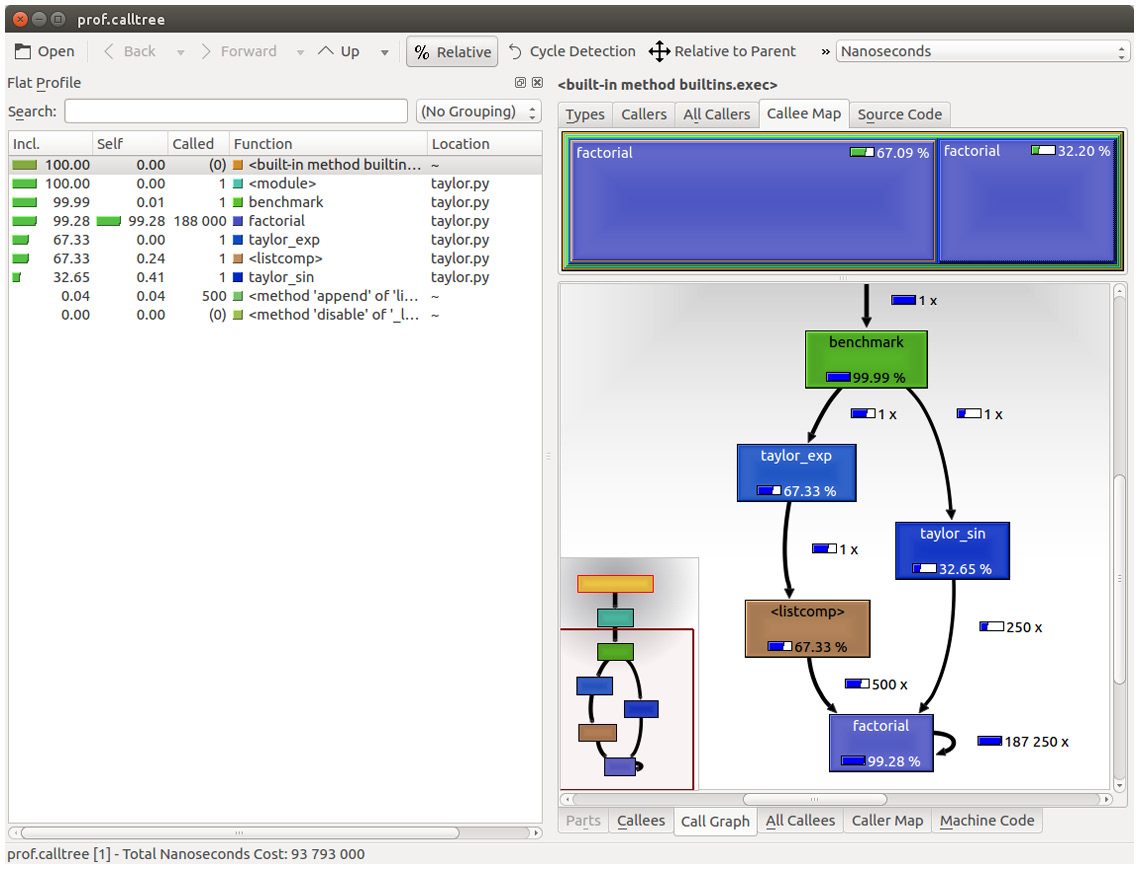Figure 1.6 – Profiling output generated by pyprof2calltree and displayed by KCachegrind

The screenshot shows the KCachegrind UI. On the left, we have an output fairly similar to `cProfile`. The actual column names are slightly different: Incl. translates to the `cProfile` module's `cumtime` value and Self translates to `tottime`. The values are given in percentages by clicking on the Relative button on the menu bar. By clicking on the column headers, you can sort them by the corresponding property.

On the top right, a click on the Callee Map tab will display a diagram of the function costs. In the diagram shown in Figure 1.6, the time percentage spent by the function is proportional to the area of the rectangle. Rectangles can contain sub-rectangles that represent subcalls to other functions. In this case, we can easily see that there are two rectangles for the `factorial` function. The one on the left corresponds to the calls made by `taylor_exp` and the one on the right to the calls made by `taylor_sin`.

On the bottom right, you can display another diagram, a call graph, by clicking on the Call Graph tab. A call graph is a graphical representation of the calling relationship between the functions; each square represents a function and the arrows imply a calling relationship. For example, `taylor_exp` calls `factorial` 500 times, and `taylor_sin` calls `factorial` 250 times. KCachegrind also detects recursive calls: `factorial` calls itself 187250 times.

You can navigate to the Call Graph or the Callee Map tab by double-clicking on the rectangles; the interface will update accordingly, showing that the timing properties are relative to the selected function. For example, double-clicking on `taylor_exp` will cause the graph to change, showing only the contribution of `taylor_exp` to the total cost.

Gprof2Dot (https://github.com/jrfonseca/gprof2dot) is another popular tool used to produce call graphs. Starting from output files produced by one of the supported profilers, it will generate a `.dot` diagram representing a call graph.

## Profiling line by line with line_profiler

Now that we know which function we have to optimize, we can use the `line_profiler` module that provides information on how time is spent in a line-by-line fashion. This is very useful in situations where it's difficult to determine which statements are costly. The `line_profiler` module is a third-party module that is available on PyPI and can be installed by following the instructions at https://github.com/rkern/line_profiler.

In order to use `line_profiler`, we need to apply a `@profile` decorator to the functions we intend to monitor. Note that you don't have to import the `profile` function from another module as it gets injected into the global namespace when running the `kernprof.py` profiling script. To produce profiling output for our program, we need to add the `@profile` decorator to the `evolve` function, as follows:

```    @profile
def evolve(self, dt):
# code```

The `kernprof.py` script will produce an output file and print the result of the profiling on the standard output. We should run the script with two options, as follows:

• `-l` to use the `line_profiler` function
• `-v` to immediately print the results on screen

The usage of `kernprof.py` is illustrated in the following line of code:

`\$ kernprof.py -l -v simul.py`

It is also possible to run the profiler in an IPython shell for interactive editing. You should first load the `line_profiler` extension that will provide the `lprun` magic command. Using that command, you can avoid adding the `@profile` decorator, as illustrated in the following screenshot: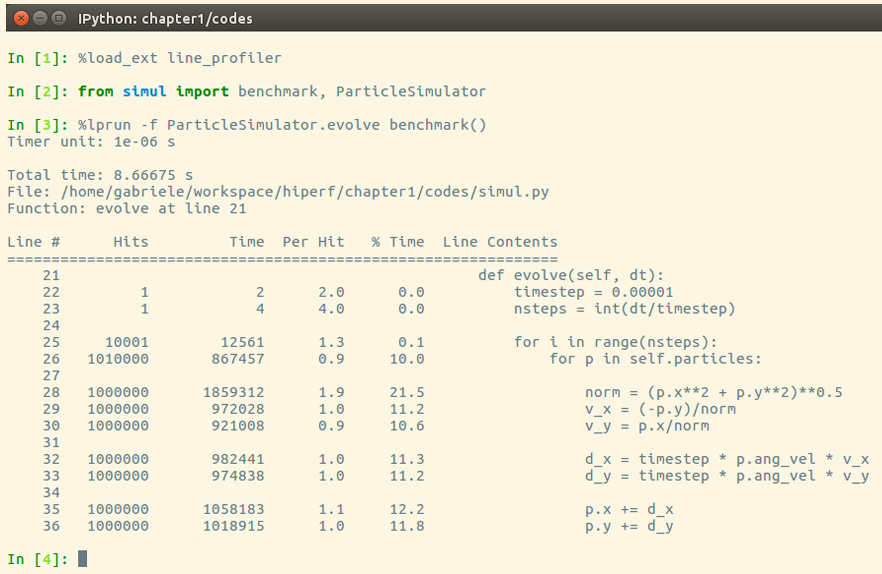Figure 1.7 – Using line_profiler within IPython

The output is quite intuitive and is divided into six columns, as follows:

• `Line #`: The number of the line that was run
• `Hits`: The number of times that line was run
• `Time`: The execution time of the line in microseconds (`Time`)
• `Per Hit`: Time/hits
• `% Time`: Fraction of the total time spent executing that line
• `Line Contents`: The content of the line

By looking at the % Time column, we can get a pretty good idea of where the time is spent. In this case, there are a few statements in the `for` loop body with a cost of around 10-20 percent each.

# Optimizing our code

Now that we have identified where exactly our application is spending most of its time, we can make some changes and assess the resulting improvement in performance.

There are different ways to tune up our pure Python code. The way that typically produces the most significant results is to improve the algorithms used. In this case, instead of calculating the velocity and adding small steps, it will be more efficient (and correct, as it is not an approximation) to express the equations of motion in terms of radius, `r`, and angle, `alpha`, (instead of `x` and `y`), and then calculate the points on a circle using the following equation:

```    x = r * cos(alpha)
y = r * sin(alpha)```

Another optimization method lies in minimizing the number of instructions. For example, we can precalculate the `timestep * p.ang_vel` factor that doesn't change with time. We can exchange the loop order (first, we iterate on particles, then we iterate on time steps) and put the calculation of the factor outside the loop on the particles.

The line-by-line profiling also showed that even simple assignment operations can take a considerable amount of time. For example, the following statement takes more than 10 percent of the total time:

`    v_x = (-p.y)/norm`

We can improve the performance of the loop by reducing the number of assignment operations performed. To do that, we can avoid intermediate variables by rewriting the expression into a single, slightly more complex statement (note that the right-hand side gets evaluated completely before being assigned to the variables), as follows:

```    p.x, p.y = p.x - t_x_ang*p.y/norm, p.y + t_x_ang *
p.x/norm```

This leads to the following code:

```        def evolve_fast(self, dt):
timestep = 0.00001
nsteps = int(dt/timestep)
# Loop order is changed
for p in self.particles:
t_x_ang = timestep * p.ang_vel
for i in range(nsteps):
norm = (p.x**2 + p.y**2)**0.5
p.x, p.y = (p.x - t_x_ang * p.y/norm,
p.y + t_x_ang * p.x/norm)```

After applying the changes, we should verify that the result is still the same by running our test. We can then compare the execution times using our benchmark, as follows:

```\$ time python simul.py # Performance Tuned
real    0m0.756s
user    0m0.714s
sys    0m0.036s
\$ time python simul.py # Original
real    0m0.863s
user    0m0.831s
sys    0m0.028s```

As you can see, we obtained only a modest increment in speed by making a pure Python micro-optimization.

# Using the dis module

In this section, we will dig into the Python internals to estimate the performance of individual statements. In the CPython interpreter, Python code is first converted to an intermediate representation, the bytecode, and then executed by the Python interpreter.

To inspect how the code is converted to bytecode, we can use the `dis` Python module (`dis` stands for disassemble). Its usage is really simple; all we need to do is call the `dis.dis` function on the `ParticleSimulator.evolve` method, like this:

```    import dis
from simul import ParticleSimulator
dis.dis(ParticleSimulator.evolve)```

This will print, for each line in the function, a list of bytecode instructions. For example, the `v_x = (-p.y)/norm` statement is expanded in the following set of instructions:

```    29           85 LOAD_FAST                5 (p)
91 UNARY_NEGATIVE
95 BINARY_TRUE_DIVIDE
96 STORE_FAST               7 (v_x)```

`LOAD_FAST` loads a reference of the `p` variable onto the stack and `LOAD_ATTR` loads the `y` attribute of the item present on top of the stack. The other instructions, `UNARY_NEGATIVE` and `BINARY_TRUE_DIVIDE`, simply do arithmetic operations on top-of-stack items. Finally, the result is stored in `v_x` (`STORE_FAST`).

By analyzing the `dis` output, we can see that the first version of the loop produces `51` bytecode instructions, while the second gets converted into `35` instructions.

The `dis` module helps discover how the statements get converted and serves mainly as an exploration and learning tool of the Python bytecode representation. For a more comprehensive introduction and discussion on the Python bytecode, refer to the Further reading section at the end of this chapter.

To improve our performance even further, we can keep trying to figure out other approaches to reduce the number of instructions. It's clear, however, that this approach is ultimately limited by the speed of the Python interpreter, and it is probably not the right tool for the job. In the following chapters, we will see how to speed up interpreter-limited calculations by executing fast specialized versions written in a lower-level language (such as C or Fortran).

# Profiling memory usage with memory_profiler

In some cases, high memory usage constitutes an issue. For example, if we want to handle a huge number of particles, we will incur a memory overhead due to the creation of many `Particle` instances.

The `memory_profiler` module summarizes, in a way similar to `line_profiler`, the memory usage of a process.

The `memory_profiler` package is also available on PyPI. You should also install the `psutil` module (https://github.com/giampaolo/psutil) as an optional dependency that will make `memory_profiler` considerably faster.

Just as with `line_profiler`, `memory_profiler` also requires the instrumentation of the source code by placing a `@profile` decorator on the function we intend to monitor. In our case, we want to analyze the `benchmark` function.

We can slightly change `benchmark` to instantiate a considerable amount (`100000`) of `Particle` instances and decrease the simulation time, as follows:

```    def benchmark_memory():
particles = [
Particle(uniform(-1.0, 1.0),
uniform(-1.0, 1.0),
uniform(-1.0, 1.0))
for i in range(100000)
]
simulator = ParticleSimulator(particles)
simulator.evolve(0.001)```

We can use `memory_profiler` from an IPython shell through the `%mprun` magic command, as shown in the following screenshot: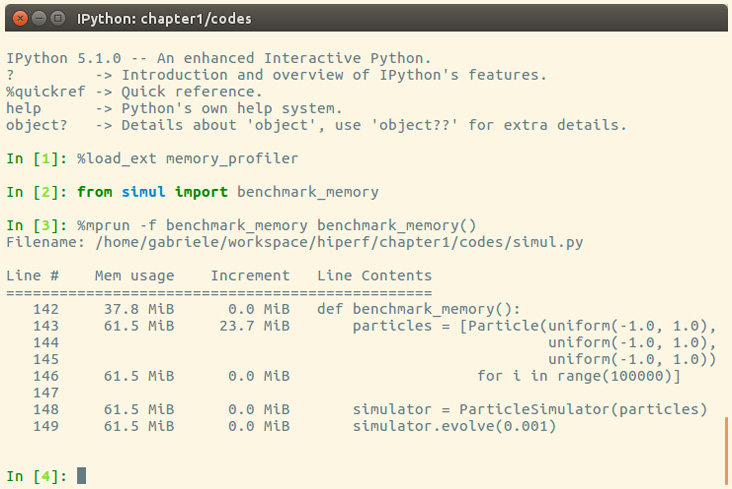Figure 1.8 – Output from memory_profiler

It is possible to run `memory_profiler` from the shell using the `mprof run` command after adding the `@profile` decorator.

From the `Increment` column, we can see that 100,000 `Particle` objects take `23.7 MiB` of memory.

1 mebibyte (MiB) is equivalent to 1,048,576 bytes. It is different from 1 megabyte (MB), which is equivalent to 1,000,000 bytes.

We can use `__slots__` on the `Particle` class to reduce its memory footprint. This feature saves some memory by avoiding storing the variables of the instance in an internal dictionary. This strategy, however, has a small limitation—it prevents the addition of attributes other than the ones specified in `__slots__`. You can see this feature in use in the following code snippet:

```    class Particle:
__slots__ = ('x', 'y', 'ang_vel')
def __init__(self, x, y, ang_vel):
self.x = x
self.y = y
self.ang_vel = ang_vel```

We can now rerun our benchmark to assess the change in memory consumption. The result is displayed in the following screenshot: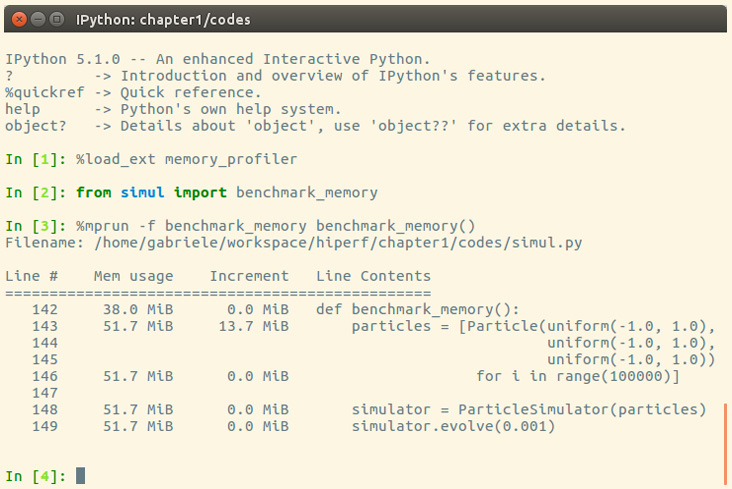Figure 1.9 – Improvement in memory consumption

By rewriting the `Particle` class using `__slots__`, we can save about 10 MiB of memory.

# Summary

In this chapter, we introduced the basic principles of optimization and applied those principles to a test application. When optimizing an application, the first thing to do is test and identify the bottlenecks in the application. We saw how to write and time a benchmark using the `time` Unix command, the Python `timeit` module, and the full-fledged `pytest-benchmark` package. We learned how to profile our application using `cProfile`, `line_profiler`, and `memory_profiler`, and how to analyze and navigate the profiling data graphically with KCachegrind.

Speed is undoubtedly an important component of any modern software. The techniques we have learned in this chapter will allow you to systematically tackle the problem of making your Python programs more efficient from different angles. Further, we have seen that these tasks can take advantage of Python built-in/native packages and do not require any special external tools.

In the next chapter, we will explore how to improve performance using algorithms and data structures available in the Python standard library. We will cover scaling and sample usage of several data structures, and learn techniques such as caching and memorization. We will also introduce Big O notation, which is a common computer science tool to analyze the running time of algorithms and data structures.

# Questions

1. Arrange the following three items in order of importance when building a software application: correctness (the program does what it is supposed to do), efficiency (the program is optimized in speed and memory management), and functionality (the program runs).
2. How could `assert` statements be used in Python to check for the correctness of a program?
3. What is a benchmark in the context of optimizing a software program?
4. How could `timeit` magic commands be used in Python to estimate the speed of a piece of code?
5. List three different types of information that are recorded and returned by `cProfile` (included as output columns) in the context of profiling a program.
6. On a high level, what is the role of the `dis` module in optimization?
7. In the `exercise.py` file, we write a simple function, `close()`, that checks whether a pair of particles are close to each other (with 1e-5 tolerance). In `benchmark()`, we randomly initialize two particles and call `close()` after running the simulation. Make a guess of what takes most of the execution time in `close()`, and profile the function via `benchmark()` using `cProfile`; does the result confirm your guess?